## *DESIGN RESPONSE

Keyword type: step

With *DESIGN RESPONSE one can define the design response functions in a sensitivity analysis. Right now the following design response functions are allowed for TYPE=COORDINATE design variables:

• ALL-DISP: the square root of the sum of the square of the displacements in all nodes of the structure or of a subset if a node set is defined
• x-DISP: the square root of the sum of the square of the x-displacements in all nodes of the structure or of a subset if a node set is defined
• Y-DISP: the square root of the sum of the square of the y-displacements in all nodes of the structure or of a subset if a node set is defined
• Z-DISP: the square root of the sum of the square of the z-displacements in all nodes of the structure or of a subset if a node set is defined
• EIGENFREQUENCY: all eigenfrequencies calculated in a previous *FREQUENCY step (actually the eigenvalues, which are the square of the eigenfrequencies)
• MASS: mass of the total structure or of a subset if an element set is defined
• STRAIN ENERGY: internal energy of the total structure or of a subset if an element set is defined
• STRESS: the maximum von Mises stress of the total structure or of a subset if a node set is defined. The maximum is approximated by the Kreisselmeier-Steinhauser function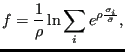(621)

where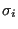is the von Mises stress in node i,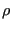and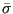are user-defined parameters. The higherthe closer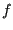is to the actual maximum (a value of 10 is recommended; the higher this value, the sharper the turns in the function).is the target stress, it should not be too far away from the actual maximum.

and for TYPE=ORIENTATION design variables:

• ALL-DISP: the displacements in all nodes.
• EIGENFREQUENCY: all eigenfrequencies (actually the eigenvalues, which are the square of the eigenfrequencies) and eigenmodes calculated in a previous *FREQUENCY step.
• GREEN: the Green functions calculated in a previous *GREEN step.
• MASS: mass of the total structure or of a subset if an element set is defined
• STRAIN ENERGY: internal energy of the total structure or of a subset if an element set is defined
• STRESS: the stresses in all nodes.

There is one parameter NAME which is compulsary for TYPE=COORDINATE design variables and not used for TYPE=ORIENTATION design variables. It is used for the sake of choosing design responses for the objective and/or constraints in an optimization. It should not be longer than 80 characters.

Exactly one *DESIGN RESPONSE keyword is required in a *SENSITIVITY step of type ORIENTATION. This keyword has to be followed by at least one design response function.

For a *SENSITIVITY step of type COORDINATE at least one *DESIGN RESPONSE keyword is required. This keyword has to be followed by exactly one design response function.

First line:

• *DESIGN RESPONSE.
• specify the parameter NAME and its value for TYPE=COORDINATE design variables.

Second line:

• an objective function
• an element or node set, if appropriate
•for the Kreisselmeier-Steinhauser function (only for the coordinates as design variables and the stress as target)
•for the Kreisselmeier-Steinhauser function (only for the coordinates as design variables and the stress as target)
Repeat this line if needed.

The design response functions STRAIN ENERGY, MASS, ALL-DISP and STRESS require a *STATIC step before the *SENSITIVITY step, the design response function EIGENFREQUENCY requires a *FREQUENCY step immediately preceding the *SENSITIVITY step and the design response function GREEN requires a *GREEN step before the *SENSITIVITY step. Therefore, the {STRAIN ENERGY, MASS, ALL-DISP, STRESS} design response functions, the {EIGENFREQUENCY} design response function and the {GREEN} design response function are mutually exclusive within one and the same *SENSITIVITY step.

Example:

*DESIGN RESPONSE
ALL-DISP,N1


defines the square root of the sum of the square of the displacements in set N1 to be the design response function.

Example files: sensitivity_I.